Newton's Laws - Lesson 4 - Newton's Third Law of Motion

# Newton's Third LawA force is a push or a pull that acts upon an object as a results of its interaction with another object. Forces result from interactions! As discussed in Lesson 2, some forces result from contact interactions (normal, frictional, tensional, and applied forces are examples of contact forces) and other forces are the result of action-at-a-distance interactions (gravitational, electrical, and magnetic forces). According to Newton, whenever objects A and B interact with each other, they exert forces upon each other. When you sit in your chair, your body exerts a downward force on the chair and the chair exerts an upward force on your body. There are two forces resulting from this interaction - a force on the chair and a force on your body. These two forces are called action and reaction forces and are the subject of Newton's third law of motion. Formally stated, Newton's third law is:

For every action, there is an equal and opposite reaction.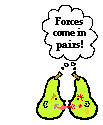The statement means that in every interaction, there is a pair of forces acting on the two interacting objects. The size of the forces on the first object equals the size of the force on the second object. The direction of the force on the first object is opposite to the direction of the force on the second object. Forces always come in pairs - equal and opposite action-reaction force pairs.

### Examples of Interaction Force Pairs

A variety of action-reaction force pairs are evident in nature. Consider the propulsion of a fish through the water. A fish uses its fins to push water backwards. But a push on the water will only serve to accelerate the water. Since forces result from mutual interactions, the water must also be pushing the fish forwards, propelling the fish through the water. The size of the force on the water equals the size of the force on the fish; the direction of the force on the water (backwards) is opposite the direction of the force on the fish (forwards). For every action, there is an equal (in size) and opposite (in direction) reaction force. Action-reaction force pairs make it possible for fish to swim.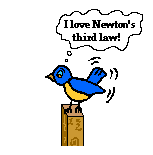Consider the flying motion of birds. A bird flies by use of its wings. The wings of a bird push air downwards. Since forces result from mutual interactions, the air must also be pushing the bird upwards. The size of the force on the air equals the size of the force on the bird; the direction of the force on the air (downwards) is opposite the direction of the force on the bird (upwards). For every action, there is an equal (in size) and opposite (in direction) reaction. Action-reaction force pairs make it possible for birds to fly.

Consider the motion of a car on the way to school. A car is equipped with wheels that spin. As the wheels spin, they grip the road and push the road backwards. Since forces result from mutual interactions, the road must also be pushing the wheels forward. The size of the force on the road equals the size of the force on the wheels (or car); the direction of the force on the road (backwards) is opposite the direction of the force on the wheels (forwards). For every action, there is an equal (in size) and opposite (in direction) reaction. Action-reaction force pairs make it possible for cars to move along a roadway surface.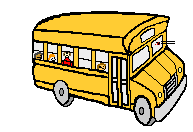1. While driving down the road, a firefly strikes the windshield of a bus and makes a quite obvious mess in front of the face of the driver. This is a clear case of Newton's third law of motion. The firefly hit the bus and the bus hits the firefly. Which of the two forces is greater: the force on the firefly or the force on the bus?

2. For years, space travel was believed to be impossible because there was nothing that rockets could push off of in space in order to provide the propulsion necessary to accelerate. This inability of a rocket to provide propulsion is because ...

a. ... space is void of air so the rockets have nothing to push off of.

b. ... gravity is absent in space.

c. ... space is void of air and so there is no air resistance in space.

d. ... nonsense! Rockets do accelerate in space and have been able to do so for a long time.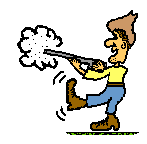3. Many people are familiar with the fact that a rifle recoils when fired. This recoil is the result of action-reaction force pairs. A gunpowder explosion creates hot gases that expand outward allowing the rifle to push forward on the bullet. Consistent with Newton's third law of motion, the bullet pushes backwards upon the rifle. The acceleration of the recoiling rifle is ...

a. greater than the acceleration of the bullet.

b. smaller than the acceleration of the bullet.

c. the same size as the acceleration of the bullet.

4. In the top picture (below), Kent Budgett is pulling upon a rope that is attached to a wall. In the bottom picture, Kent is pulling upon a rope that is attached to an elephant. In each case, the force scale reads 500 Newton. Kent is pulling ...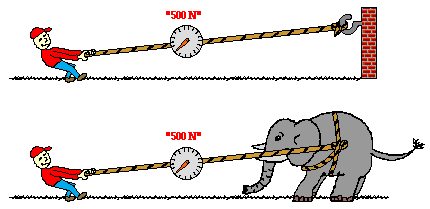a. with more force when the rope is attached to the wall.

b. with more force when the rope is attached to the elephant.

c. the same force in each case.

Next Section: